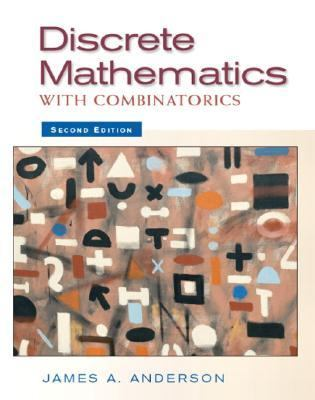118265

9780130457912

# Discrete Mathematics With Combinatorics\$5.30
\$3.95 Shipping
List Price
\$122.20
Discount
95% Off
You Save
\$116.90

• Condition: Acceptable
• Provider: GoodwillTucson Contact
• Provider Rating:
88%
• Ships From: Tucson, AZ
• Shipping: Standard, Expedited
• Comments: Used book in acceptable condition. Has some wear to binding, spine, cover, and/or pages. Some markings/writing may be present. May have some stickers and/or sticker residue present. Pages have water stains and/or some wavy pages. If applicable, book's access code, disc, and/or accessories are not included unless otherwise specified. All items shipped Monday - Friday. Fast shipping - Books ship in envelope.30-day money back guarantee

Most renters respond to questions in 48 hours or less.
The response will be emailed to you.
• ISBN-13: 9780130457912
• ISBN: 0130457914
• Edition: 2
• Publication Date: 2003
• Publisher: Prentice Hall PTR

### AUTHOR

Anderson, James A., Lewis, Jerome

### SUMMARY

As in the first edition, the purpose of this book is to present an extensive range and depth of topics in discrete mathematics and also work in a theme on how to do proofs. Proofs are introduced in the first chapter and continue throughout the book. Most students taking discrete mathematics are mathematics and computer science majors. Although the necessity of learning to do proofs is obvious for mathematics majors, it is also critical for computer science students to think logically. Essentially, a logical bug-free computer program is equivalent to a logical proof. Also, it is assumed in this book that it is easier to use (or at least not misuse) an application if one understands why it works. With few exceptions, the book is self-contained. Concepts are developed mathematically before they are seen in an applied context. Additions and alterations in the second edition: More coverage of proofs, especially in Chapter 1. Added computer science applications, such as a greedy algorithm for coloring the nodes of a graph, a recursive algorithm for counting the number of nodes on a binary search tree, or an efficient algorithm for computinga b modnfor very large values ofa, b,andn. An extensive increase in the number of problems in the first eight chapters. More problems are included that involve proofs. Additional material is included on matrices. Inclusion of finite states with output and Turing machines. True-False questions at the end of each chapter. Summary questions at the end of each chapter. A glossary at the end of each chapter. Functions and sequences are introduced earlier (in Chapter 2). Calculus is not required for any of the material in this book. College algebra is adequate for the basic chapters. However, although this book is self-contained, some of the remaining chapters require more mathematical maturity than do the basic chapters, so calculus is recommended more for giving maturity, than for any direct uses. This book is intended for either a one- or two-term course in discrete mathematics. The first eight chapters of this book provide a foundation in discrete mathematics and would be appropriate for a first-level course for freshmen or sophomores. These chapters are essentially independent, so that the instructor can pick the material he/she wishes to cover. The remainder of the book contains appropriate material for a second course in discrete mathematics. These chapters expand concepts introduced earlier and introduce numerous advanced topics. Topics are explored from different points of view to show how they may be used in different settings. The range of topics include: Logic--Including truth tables, propositional logic, predicate calculus, circuits, induction, and proofs. Set Theory--Including cardinality of sets, relations, partially ordered sets, congruence relations, graphs, directed graphs, and functions. Algorithms--Including complexity of algorithms, search and sort algorithms, the Euclidean algorithm, Huffman's algorithm, Prim's algorithms, Warshall's algorithm, the Ford-Fulkerson algorithm, the Floyd-Warshall algorithm, and Dijkstra's algorithms. Graph Theory--Including directed graphs, Euler cycles and paths, Hamiltonian cycles and paths, planar graphs, and weighted graphs. Trees--including binary search trees, weighted trees, tree transversal, Huffman's codes, and spanning trees. Combinatorics--including permutations, combinations, inclusion-exclusion, partitions, generating functions, Catalan numbers, Sterling numbers, Rook Polynomials, derangements, and enumeration of colors. Algebra--Including semigroups, groups, lattices, semilattices, Boolean algebras, rings, fields, integral domains,Anderson, James A. is the author of 'Discrete Mathematics With Combinatorics', published 2003 under ISBN 9780130457912 and ISBN 0130457914.

You can find lots of answers to common customer questions in our FAQs

View a detailed breakdown of our shipping prices